# Using Counters in SPIN

Each counter controls two pins, each COG has two counters, each Propeller has eight COGs, how many HATs were going to St Ives?

While the Parallax Propeller keeps hardware features to a minimum, it makes room for counters. Lots of them, in fact. The Propeller has two counters in each of its 8 COGs, making a total of 16. Each of these counters can control 2 IO pins- so that's all of the Propeller's 32 IO pins covered.

## Anatomy of a Counter

Each counter has a set of registers that govern how it behaves, they are:

• `PHSx` - where your counter value is accumilated. It can be read/written like any other variable.
• `FRQx` - the value that's accumilated into PHSx.
• `CTRx` - governs how the counter behaves and which IO pins it's associated with.

Each of these registers has an A and B counterpart- ie: PHSA/PHSB to differentiate the two counters on each COG. The most complicated and important registers are CTRA and CTRB. These are the control registers for each counter and determine whether the counter is enabled, which pins it's associated with and how it behaves.

Comparatively FRQA/FRQB and PHSA/PHSB are very simple, FRQx is simply a number that can be added to the value of PHSx under certain conditions as defined in CTRx.

## Understanding the Control Register

As all registers on the Propeller consist of 32 bits, the control register is simply a series of 32 1s or 0s which are grouped together to configure the 4 options available: Mode, PLL Divider, Pin B and Pin A.

### Mode

The table below shows the available values for Mode, which occupies bits 30 to 26 of the CTRx register:You can set these bits easily in SPIN, like so:

``````CTRA[30..26] = %11111
``````

Or if you're more familiar with bit shifting, like so:

``````CTRA := %11111 << 26
``````

### PLL Divider

The PLL divider only affects the modes marked PLL in the counter modes table. The PLL is given the output of `PHSx` ( bit 31 of the Phase register ) as its clock source, and multiplies it by 16. The PLL divider settings allow you to take the resulting clock and divide it by 1, 2, 4, 8, 16, 32, 64 or 128.

• `%000` = Input Clock * 16 / 128 or 1/8 Input Clock
• `%001` = Input Clock * 16 / 64 or 1/4 Input Clock
• `%010` = Input Clock * 16 / 32 or 1/2 Input Clock
• `%011` = Input Clock * 16 / 16 or Input Clock * 1
• `%100` = Input Clock * 16 / 8 or Input Clock * 2
• `%101` = Input Clock * 16 / 4 or Input Clock * 4
• `%110` = Input Clock * 16 / 2 or Input Clock * 8
• `%111` = Input Clock * 16 / 1 or Input Clock * 16

Pins are then toggled at the output frequency of the PLL.

There are some caveats with this approach, however; the PLL can only operate at frequencies from 4Mhz to 8Mhz and will deliver output frequencies of 500kHz to 128Mhz. The absolute minimum input frequency is 250Hz. You cannot, therefore, use it to blink an LED every second at 1Hz.

Like the Mode, you can set the Divider like so:

``````CTRA[25..23] := %111
``````

Or like so:

``````CTRA := %111 << 23
``````

However, if you set it like this, make sure you also set the Mode at the same time since you'll overwrite it with zeros otherwise!

``````CTRA := %111 << 23                     ' Will reset Mode
CTRA := (%11111 << 26) | (%111 << 23) ' Will preserve Mode
``````

## Pin A and B

In some cases you don't need to set Pin A or B, but if you're using some of the LOGIC modes that the counter supports then you'll almost cerainly want to set at least one. A logic mode like `A == B` will, for example only increment the value of `PHSx` by adding to it the value of `FRQx` if Pin A is equal to Pin B.

When choosing your pins, refer to the Counter Modes table to figure out which ones you need- the second counter mode involves no pin states at all, and neither do `%10000` "never", or `\$11111` "always" which will either never increment `PHSx` or increment it on every clock cycle respectively.

``````CTRA[5..0]  := 0 ' Set pin A to 0
CTRA[14..9] := 1 ' Set pin B to 1
``````

When using counter modes that output something to the pin, you must make sure that you configure that pin as an output using the direction register. This applies for each COG, they must have their pins configured as outputs individually:

``````DIRA := 1 ' Set pin 0 to an output
DIRA := 1 ' Set pin 1 to an output
``````

If you fail to do this, your counter will run absolutely fine, but the output pin(s) will never change!

## Putting it all together

Now we're going to assemble what we've learned into a working example- once again we'll use blinking LEDs since they're reliable and readily available.

This example will set up `CTRA` and `CTRB` with the same settings. This should cause our LEDs to both blink around once a second. Not exactly once a second, but close enough for a demonstration:

``````CON
_CLKMODE = xtal1 + pll16x
_XINFREQ = 6_000_000

LED_A = 0
LED_B = 1
LED_C = 2

{{
(2^32) / 96_000_000 = 44.7392426667
This means that adding 44 to PHSx every clock cycle
will toggle bit 31 approximately every 0.5 seconds
}}

PUB Main
DIRA[LED_A] := 1
DIRA[LED_B] := 1
DIRA[LED_C] := 1

PHSA := 0
PHSB := 0

'         Mode             Divider        Pin B      Pin A
CTRA := (%00100 << 26) + (%000 << 23) + (0 << 9) + LED_A
FRQA := 22

'         Mode             Divider        Pin B      Pin A
CTRB := (%00100 << 26) + (%000 << 23) + (0 << 9) + LED_B
FRQB := 22

repeat ' Actual 1sec blinking LED for comparison
OUTA[LED_C] := !OUTA[LED_C]
waitcnt(clkfreq + cnt)
``````

So, what's going on here? Well, our clock frequency is 96Mhz, or 96,000,000. This happens to fit into a 32bit integer about 44 times. This means that if we add 44 to our `PHSA` register every clock tick, it will overflow approximately once every second and loop back around.

``````Size of 32bit     Clock Ticks/Second
(2^32)         /  95_000_000 = 44.7392426667
``````

Note that the actual number we need to accumilate every clock tick to overflow every second is 44.7392426667- we can't represent this floating point number in our 32bit `FRQx` registers, so we just have to go with a whole number instead.

The actual LED, or output pin, is driven by bit 31 of `PHSA`. This is the most significant bit, and it will be 0 during the first half of the accumilation, and 1 during the second. This means if you filled it at a rate of 44 per clock tick it would be 0 for about 0.5 seconds, and 1 for about 0.5 seconds giving us a blink frequency of 0.5 seconds.

To blink once every 1 second, we can divide our overflow rate by 2, `44 / 2 = 22`. This isn't very accurate, if you connect a third LED up to pin A2 you will see that the counter LED gets very slowly out of phase with the LED using `waitcnt`.

### Phase Shift

What if we wanted our LEDs to blink slightly out of phase with each other? The `PHSx` register isn't called "phase" by accident! If you give it an initial value then it will place the counter out of phase with another counter using the same settings. Try setting `PHSA` to `CLKFREQ * 22` and see what happens!

## Others Modes And Methods

Let's try another counter mode- differential `\$00101` - which will turn on either one pin, or the other. I'll also use this opportunity to demonstrate the alternative method of setting these registers:

``````CON
_CLKMODE = xtal1 + pll16x
_XINFREQ = 6_000_000

LED_A = 0
LED_B = 1

PUB Main
DIRA[LED_A] := 1
DIRA[LED_B] := 1

PHSA := 0

CTRA[30..26] := %00101 ' Mode
CTRA[5..0]   := 0      ' Pin A
CTRA[14..9]  := 1      ' Pin B
CTRA[25..23] := %000   ' Divider
FRQA := 45

repeat ' Keep COG alive
``````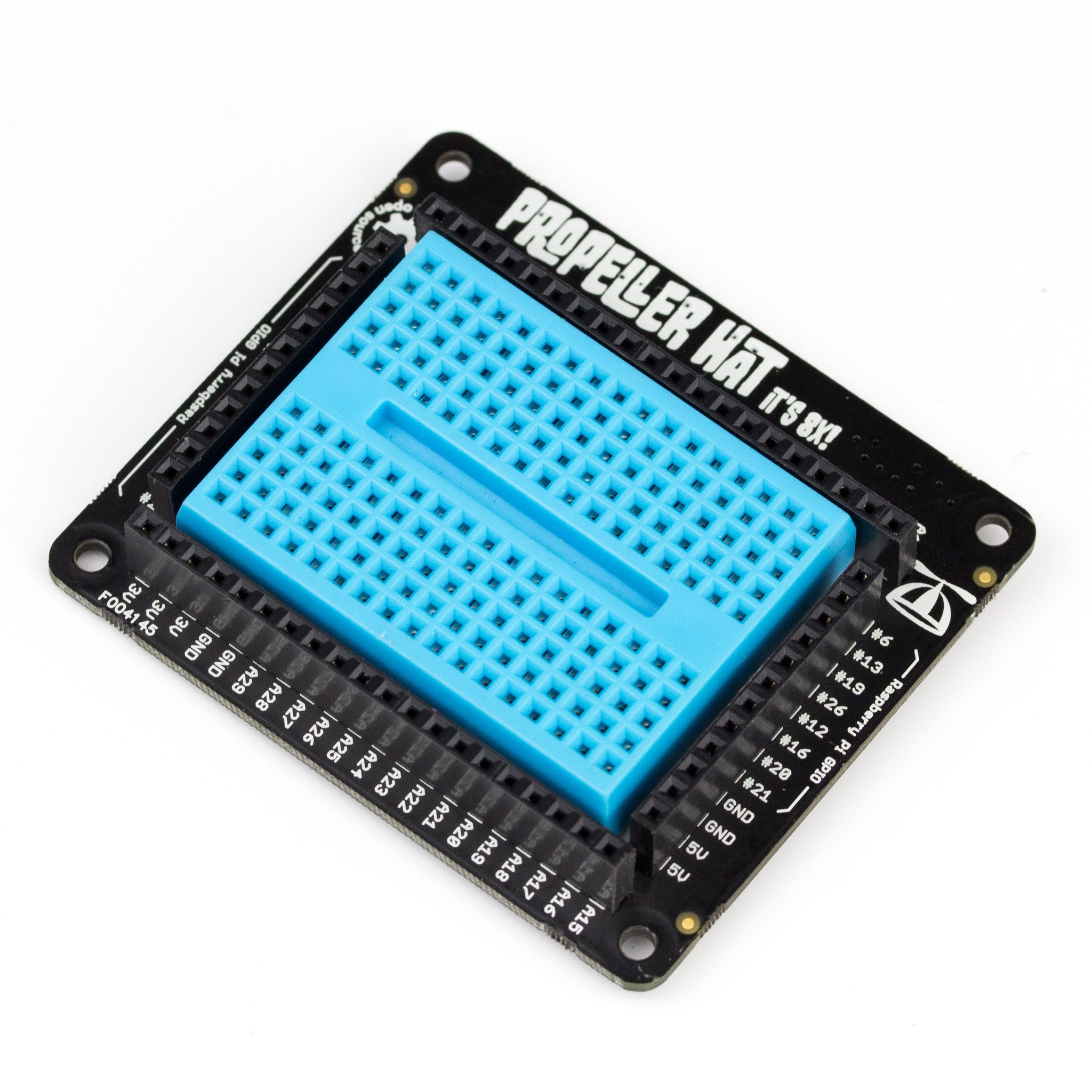Propeller HAT £15.00LED - 5mm - pack of 10 Red £1.00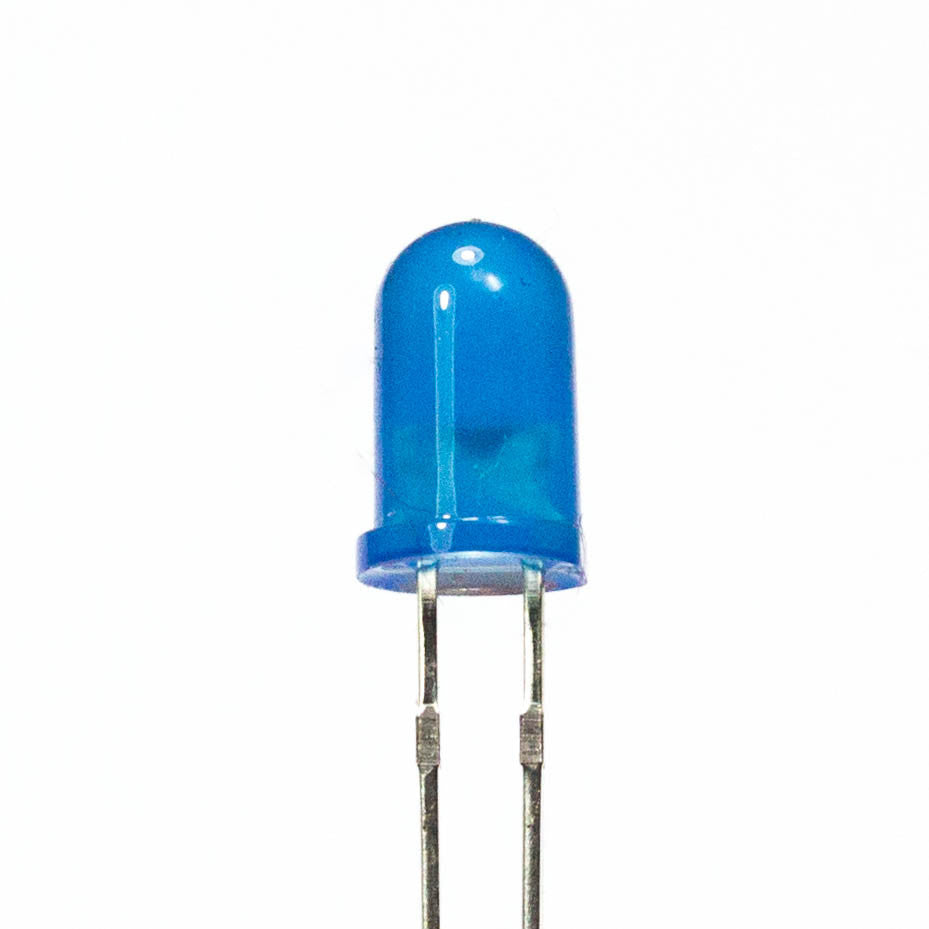LED - 5mm - pack of 10 Blue £1.00LED - 5mm - pack of 10 Green £1.00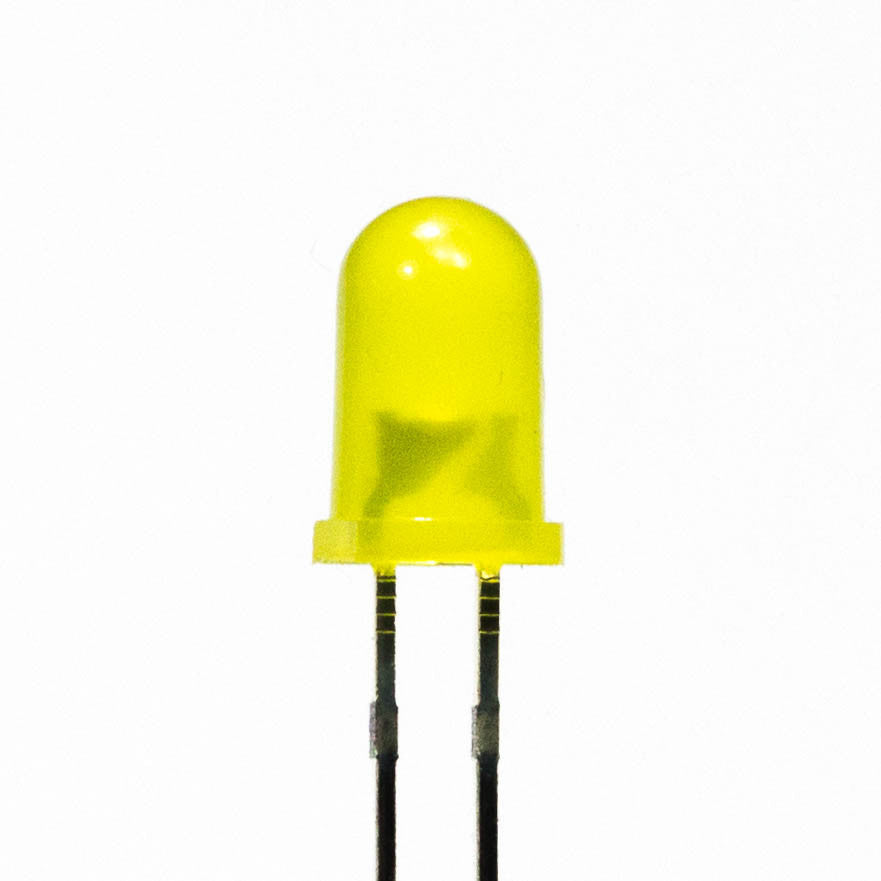LED - 5mm - pack of 10 Yellow £1.00Jumper Jerky Junior Male to Male £2.50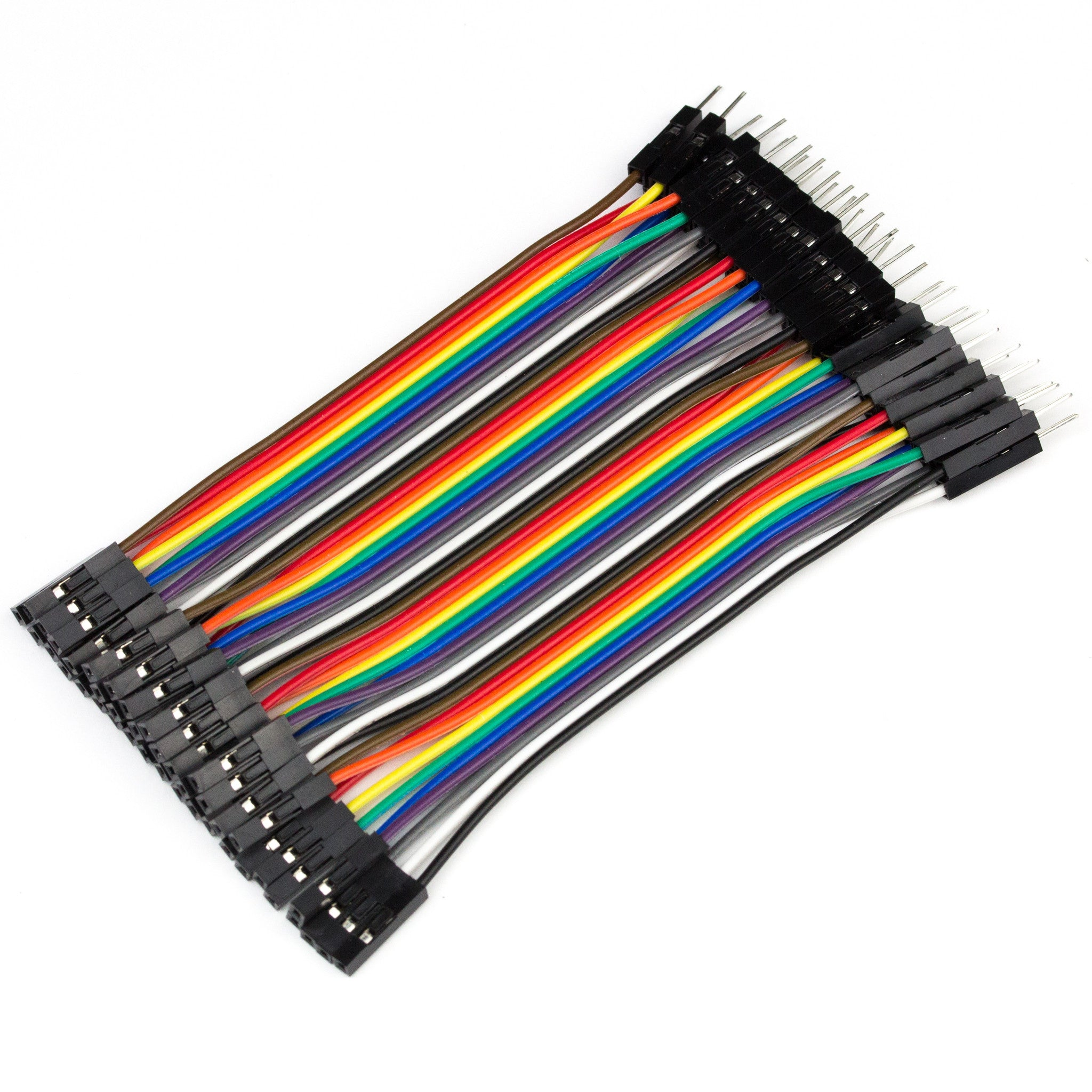Jumper Jerky Junior Male to Female £2.50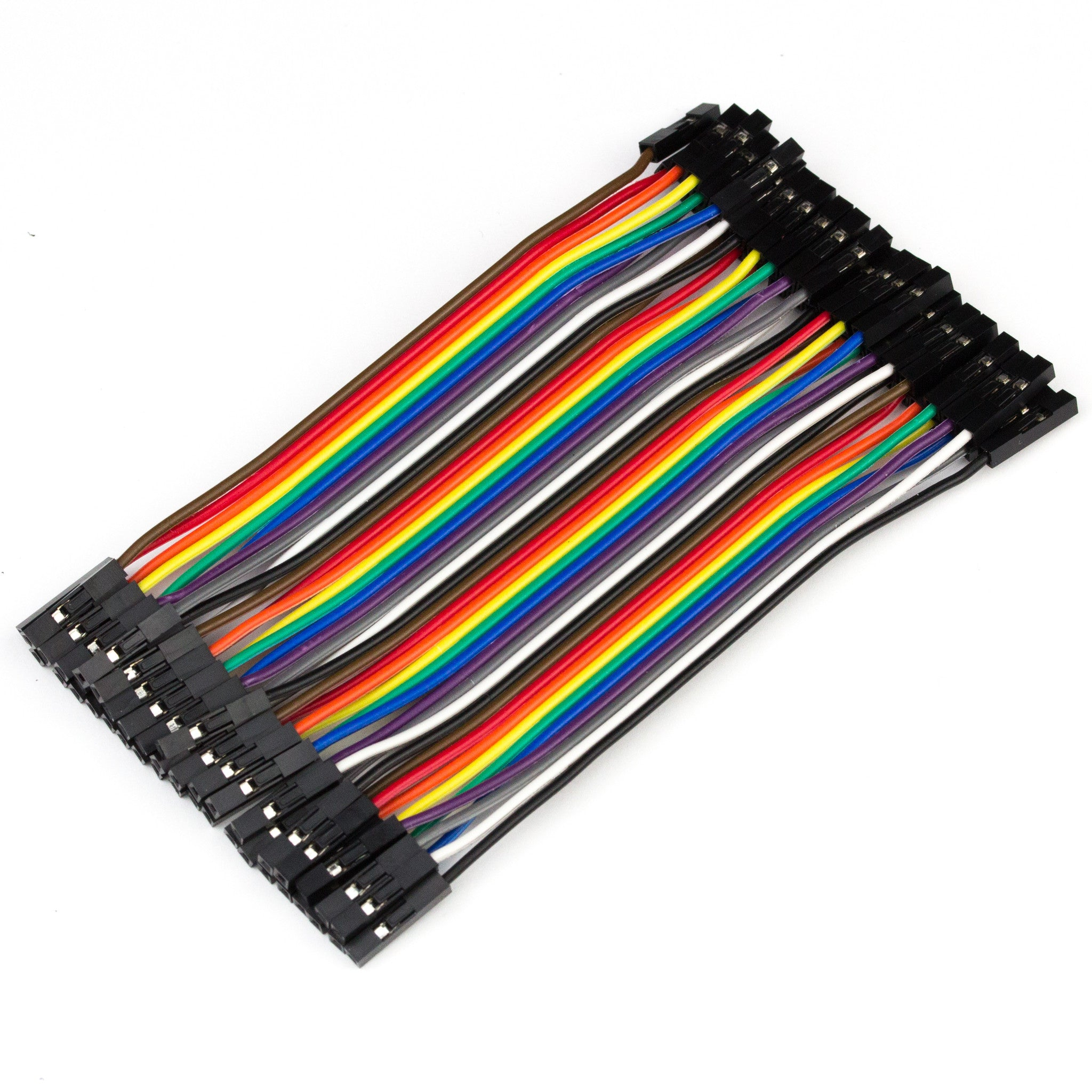Jumper Jerky Junior Female to Female £2.50Phil is Pimoroni's software guru, instantly recognisable by his somewhat pirate-themed moustache growing attempts. Usually found buried neck deep in Python libraries, he's also been known to escape on occasion and turn out crazy new products. If you need a helping hand, he's a prolific Twitter user and rampages around the forums like a T-Rex. Ask him if you need help with Pimoroni's software libraries, or Propeller HAT.